Wire D

Wire length 1 m is bent so that it forms a semicircle circuit (including the diameter). Determine the radius of the semicircle.

Result

r =  0.19 m

Solution:Leave us a comment of example and its solution (i.e. if it is still somewhat unclear...):Be the first to comment!To solve this example are needed these knowledge from mathematics:

Do you want to convert length units?

Next similar examples:

1. Estate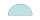Semicircle estate must be fence. The straight section has 26 meters long fence. How many meters of fence should buy?
2. Athlete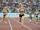How long length run athlete when the track is circular shape of radius 120 meters and an athlete runs five times in the circuit?
3. Circle r,DCalculate the diameter and radius of the circle if it has length 52.45 cm.
4. BaseBase of building is circle with diameter 25 m. Calculate the circumference of a circular trench witch diameter is 41 cm wider than the diameter of the base.
5. Wheel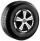What is the wheel diameter if on the 0.38 km track turns 128 times?
6. Meridian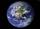What is the distance (length) the Earth's meridian 23° when the radius of the Earth is 6370 km?
7. Two gears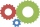The two gears fit together. The larger gear has 32 teeth, the smaller has 20 teeth less. How many times does turn a smaller gear if the bigger gear turns three times?
8. ThalesCalculate the length of the Thales' circle described to right triangle with hypotenuse 18.4 cm.
9. Average height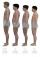In a class are 34 students. The average height of students is 165 cm. What will be the average height of students in the classroom when two pupils tall 176 cm and 170 cm moved from this school/class?
10. MineWheel in traction tower has a diameter 5 m. How many meters will perform an elevator cabin if wheel rotates in the same direction 49 times?
11. FlowerbedIn the park there is a large circular flowerbed with a diameter of 12 m. Jakub circulated him ten times and the smaller Vojtoseven times. How many meters each went by and how many meters did Jakub run more than Vojta?
12. Silo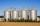Outer perimeter of silo is 32 m. Concrete wall is 35 cm thick. What is diameter and area of inside floor of silo?
13. Wheels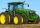Small tractor wheel with a diameter of 60 cm must be rotated 8 times to overcome some pathway. How many times must turn the big tractor wheel with a radius 60 cm to overcome the same distance?
14. Wheel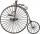Diameter of motocycle wheel is 52 cm. How many times rotates wheel on roand long 2 km?
15. CircleWhat is the radius of the circle whose perimeter is 6 cm?Determine the radius of the circle, if its perimeter and area is the same number.We want to prove the sentence: If the natural number n is divisible by six, then n is divisible by three. From what assumption we started?